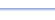Program: DIST3D

DIST3D

• Finds the distance diagonally across a rectangular prism, also known as the length of the diagonal of the rectangular prism, also known as the distance in 3 dimensions.

Useful for:

• SAT
• ACT
• Geometry
• Precalculus
• Calculus

See full list of programs

Try these problems using DIST3D:
on MathPro Q&AExample

Q: The figure at the
right is a rectangular
prism.  What is the
length of the green
segment?

Solution:

Run DIST3D.  How?

Enter 4, 5, and 6
for the lengths.

The program reports:

Interpretation:  The length of the diagonal is 77, or approximately 8.8.